# Electronics and Communication Engineering - Electromagnetic Field Theory

41.

In case of static electric field Maxwell's divergence equation states that:

 A. ∇ .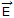= 1/r B. ∇ .= r/ε0 C. ∇ .= εr D. ∇ .= r

Explanation:

No answer description available for this question. Let us discuss.

42.

For a reflection co-efficient of 0.5, the value of SWR is

 A. -1 B. 1 C. 2 D. 3

Explanation: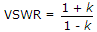.

43.

A rectangular waveguide having TE10 mode as dominant mode is having a cut off frequency of 18 GHz for the TE30 mode. The inner broad wall dimension of the rectangular waveguide is

 A. 5/3 cm B. 5 cm C. 5/2 cm D. 10 cm

Explanation: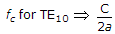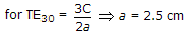44.

A 50 p characteristic impedance line is connected to a load which shows a reflection coefficients of 0.268. If Vin = 15 volt, then the net power delivered to the load will be

 A. 0.139 W B. 1.39 W C. 0.278 W D. 2.78 W

Explanation:

r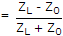, P = v2/ZL.

45.

1 dB is equal to __________ nepier

 A. 8.6 B. 6.8 C. 8 D. 0